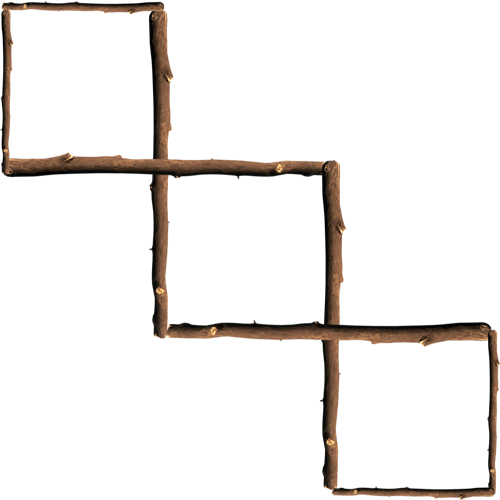Riddle: What do mathematics teachers like to eat?
Riddle: What happened to the plant in the math classroom?
Answer: It grew square roots, of course.
Riddle: What do you call two math friends?
Riddle: What three whole, positive numbers have the same answer when multiplied together as when added together?
Answer: 1, 2 and 3.  1 x 2 x 3 = 1 + 2 + 3 = 6.
Riddle: What did one math book say to another?
Answer: I have so many problems.
Riddle: Take eight small sticks, four of which are half the length of the other four. How can you make three equal squares out of the sticks?
Answer: Use the longer four sticks to be sharing sides between the squares and at the end their should be three intertwined squares.Riddle: What is the next number in the series? 7,645 5,764 4,576...
Answer: 6,457. The last digit is moved to the front to make the next number.